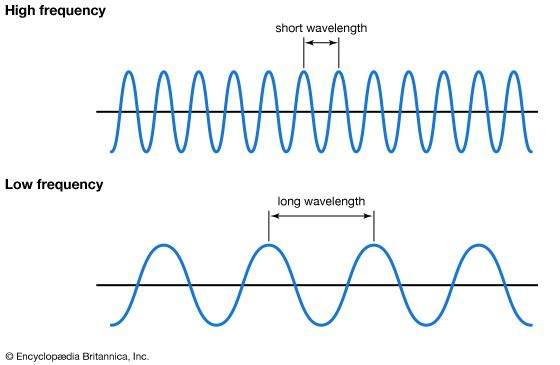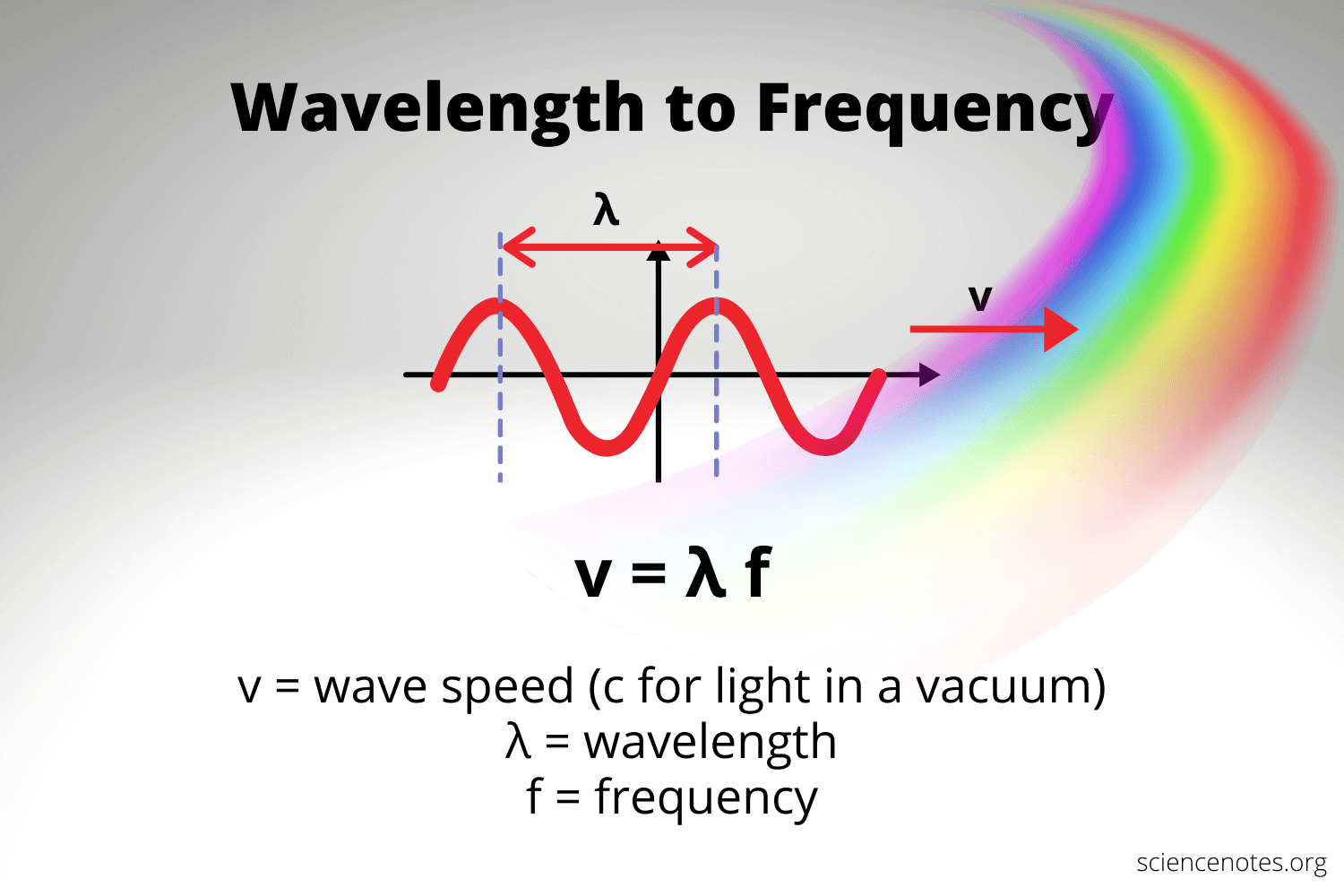# Wavelength and Frequency Study Guide

INTRODUCTION

The study of wavelength and frequency of waveforms are crucial concepts of physics and engineering. The relevant studies help to find out the relationship between them and eventually solve the required numerical.

### FREQUENCY

The number of times an entire waveform passes a particular point in a specified period is called the frequency of the waveform. The frequency unit is Hz, which is the number of waveforms that recur in one second. The letters f and the Greek letters nu (ν) is the most commonly used symbols indicating frequency.

### WAVELENGTH

The distance between two consecutive crests or troughs of consecutive oscillations of a wave is called the wavelength of that particular wave. Both traveling and stationary waves have this property. It is measured in terms of distance such as mm, cm, meters, and so on and is represented by lambda (λ).Source

### CALCULATION OF FREQUENCY AND WAVELENGTH

The wavelength of a wave is the ratio of the velocity of the wave with which it travels and the frequency of the wave. The frequency wavelength formula is given by

``                               λ = 𝜈/f``

Where 𝜈 is the velocity of the waveform, if only the value of the frequency is given and the velocity is unknown, the value of the velocity of light in a vacuum is generally taken while calculating the wavelength of the wave.Let us answer some frequently asked questions.Source

### FAQs

1. How do you calculate wavelength when given frequency?

The wavelength of a wave is the ratio of the velocity of the wave with which it travels and the frequency of the wave. The frequency wavelength formula is given by: λ = 𝜈/f

where 𝜈 is the velocity of the waveform, if only the value of the frequency is given and the velocity is unknown, the value of the velocity of light in a vacuum is generally taken while calculating the wavelength of the wave.

2. What happens when you change the wavelength and frequency?

The frequency of a wave formula can be written as

``                              f = 𝜈/ λ``

where f is the frequency of a waveform, 𝜈 is the velocity of the wave in a particular medium, and λ is the corresponding wavelength of the wave in that medium. Keeping the velocity of the waveform constant, the wavelength of the wave changes with a change in frequency.

3. What is the relationship between wavelength and frequency?

In the case of traveling waves, the wavelength of a wave is linked to its frequency and the speed at which it travels. A wave that completes more number of wave cycles in a fixed time — say 1 second is faster than its slower counterpart. Therefore, the velocity of a wave is crucial while calculating a wave’s frequency. Two different waves with two different wavelengths can have the same frequency. Two waves, Wave A and Wave B with wavelengths 1 and 3 can have the same frequency only if Wave A can travel with a speed that is 3 times the speed of Wave B. The frequency equation is shown.

``                Frequency(f) = Velocity(𝜈)/Wavelength(λ) ``

4. Are frequency and wavelength inversely related?

Yes, frequency and wavelength are inversely proportional to each other. Keeping the velocity constant, the frequency of a waveform increases with a decrease in wavelength and decreases with the increase in wavelength.

We hope you enjoyed studying this lesson and learned something cool about Wavelength and Frequency! Join our Discord community to get any questions you may have answered and to engage with other students just like you! Don’t forget to download our app to experience our fun VR classrooms – we promise it makes studying much more fun! 😎

## SOURCES

1. Frequency to Wavelength: https://www.everythingrf.com/rf-calculators/frequency-to-wavelength. Accessed 28 Feb 2022.
2. Wavelength and Frequencies Calculations: https://chem.libretexts.org/Bookshelves/Introductory_Chemistry/Introductory_Chemistry_(CK-12)/05%3A_Electrons_in_Atoms/5.02%3A_Wavelength_and_Frequency_Calculations. Accessed 28 Feb 2022.
]]>